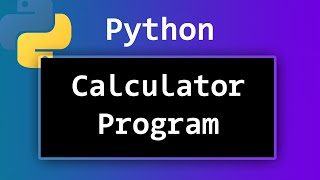## Introduction

Calculator
Photo by: Pexels, Pixabay

Mathematics and Programming are dependent on each other in many ways! The problem-solving skills involved in solving complex algebra are not dissimilar to those needed in the creation of games and applications.

Python is a text-based programming language and is very popular for its simplistic yet effective applications.

Companies such as Google and NASA use Python as Python is very good at handling complex maths (such as that needed to launch rockets into space!)

Before you start, in the Links section you can read about the History of the Calculator, there is also a short video about the Evolution of the Calculator in the video section.

Understanding how a calculator works is also useful before you program your own, in the links section you can read about How Does a Calculator Work?Photo by: Edar, Pixabay.

A Maths class has run out of calculators!

Using Python, create a console-based calculator that the class can use while they wait for their replacements.

## Process

In this project we will be making a basic calculator in python.

The program should:

• Ask the user for 2 numbers
• Print out the result

Extension

• Allow the user to choose which operation they would like to perform (+ - ÷ x )

Before you start please watch the video in the videos section How to Create a Simple Calculator so you understand what steps you will be taking.

You will need to read through "Level 1 - Arithmetic Calculator" in the documents section.

Steps to follow using the Level 1-arithmetic calculator presentation:

First open Python or use the following link for an online platform: Python Online Editor

1. On slides 8-9 follow the instructions on how to input data, store in a variable and output the contents of the variable. On slide 10 look at how to use different Mathematical Operators which will be needed for your calculator.
2. Next you need to add the two inputs from the use, read through slide 11 to understand how to complete this process.
3. Once the calculation has been completed, the next step is to output the answer to the user. Use slide 12 to assist you with this part.
4. The extension task is to allow the user to select the type of calculation, use slides 13-16 to complete this part.
5. Slide 17 shows the final code.

## Conclusion

Check you have now have completed the following Learning Outcomes:

•  Output information to the user.
•  Take inputs from the user.
•  Use IF statements.
•  Use mathematical operators.

Calculators are everywhere! Not only in Maths class, but on your phone and your computer.

Whether it’s a finance company or software on a cashier’s till, using maths is a crucial part of programming applications.

CONGRATULATIONS!

You have created your own arithmetic calculator using Python.

Next steps:

You should now move onto 2D shape calculator in Python which allows to pupils to create a calculator in Python to calculate the perimeters and areas of shapes.

## Learning Objectives

Learning Objectives

• Learn how to output information to the user.
• Learn how to take input from the user.
• Use IF statements.
• Use mathematical operators.

## Documents

Level1ArithmeticCalculator

## Videos

Evolution of the Calculator 2,000 BCE - 2020 | History of Calculator, Documentary videoHow to Create a Simple Calculator Program using Python ? Video Tutorial